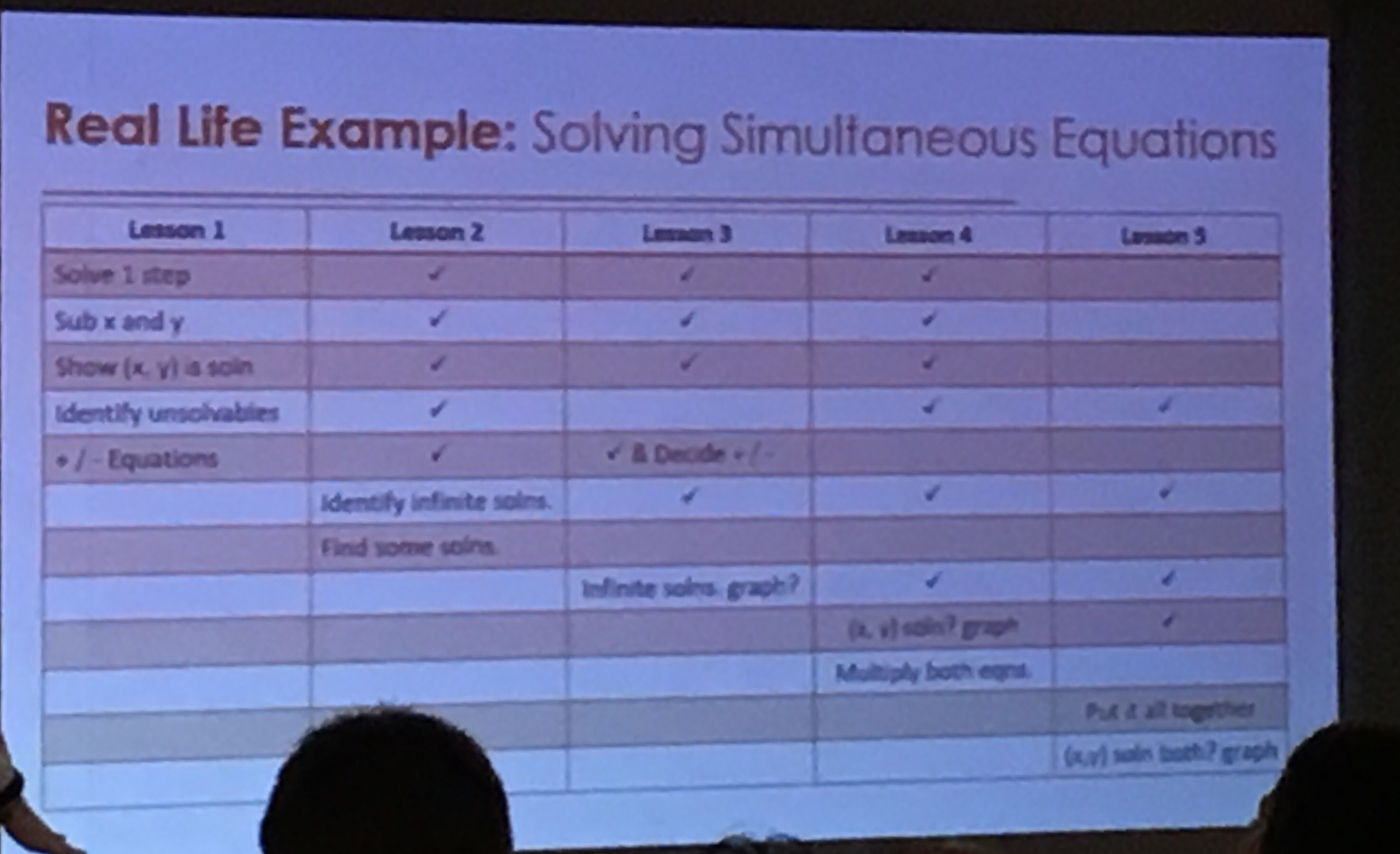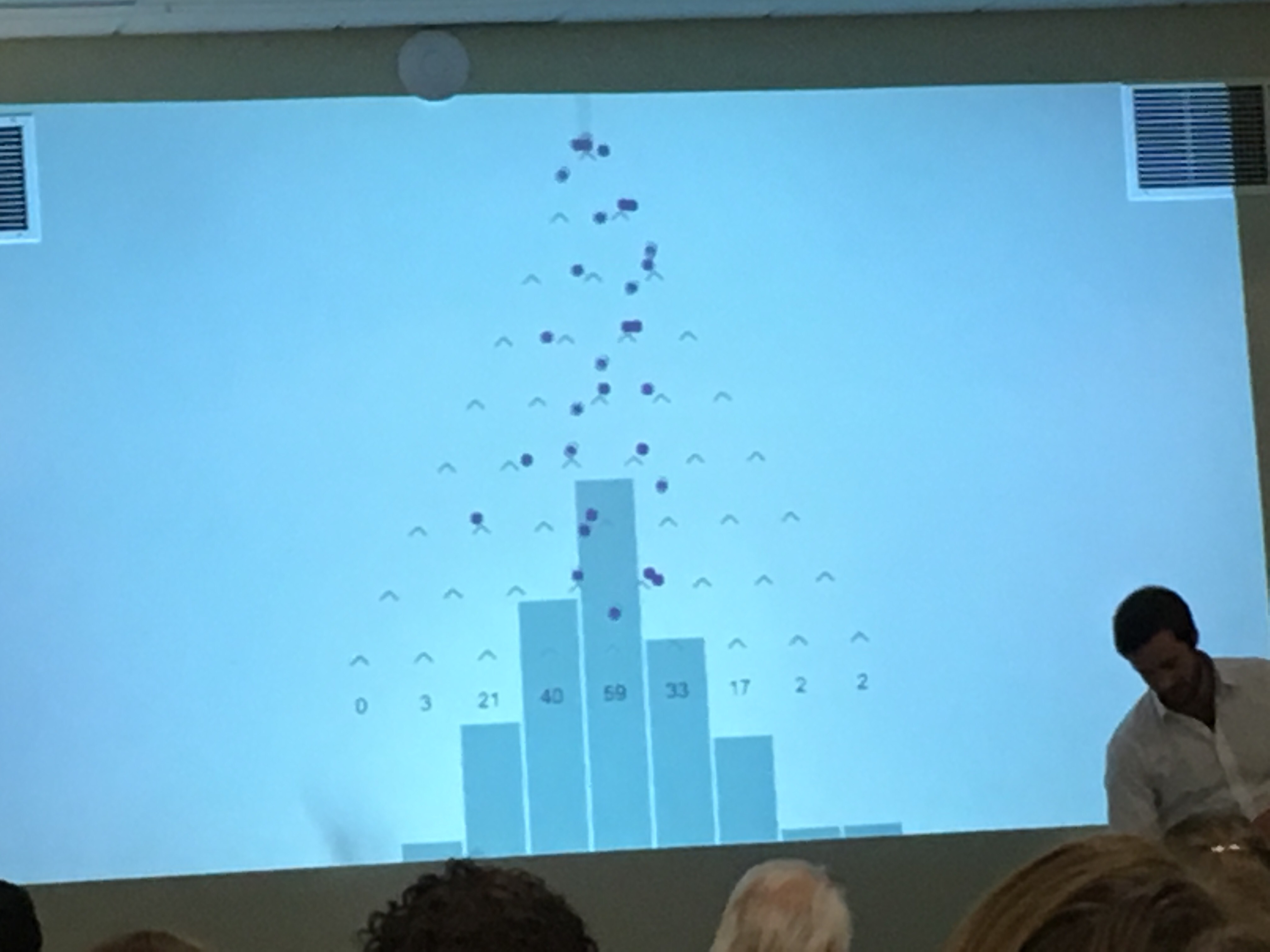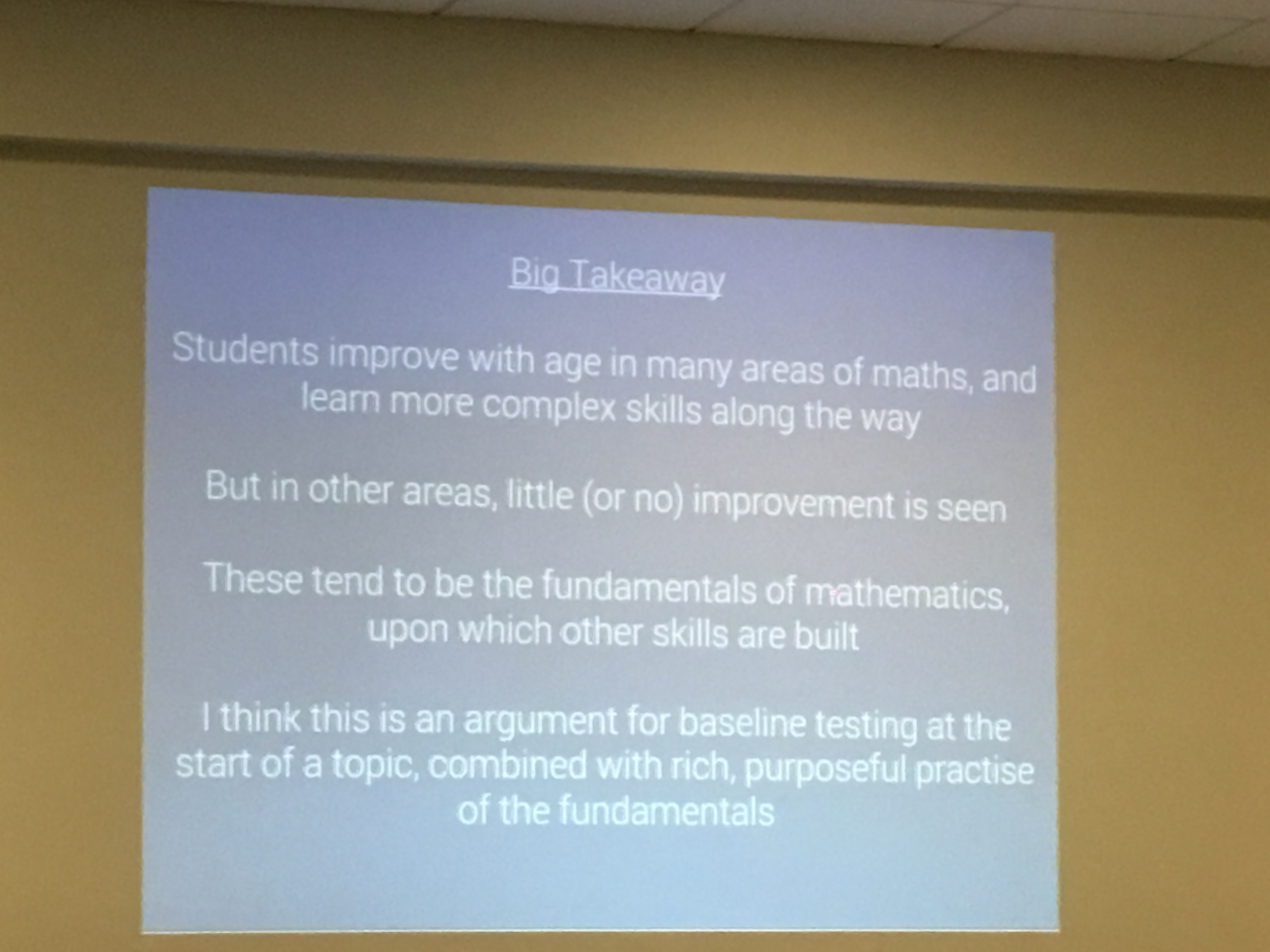Categories

# #Mathsconf8

After a very early drive to Kettering (via Leicester) I was ready for my busiest Mathsconf yet. As well as running a workshop on Geogebra, I was representing the East Midlands West MathsHub (@EM_MathsHub), and launching a new A-Level textbook for which I am one of the editors with Tarquin Group (@TarquinGroup). Because of this I didn’t manage to get to go to the opening or the speed dating segments sadly but I did manage to make the main sessions.

My descriptions below are really just the rough notes I made during the sessions (with a bit of neatening up) and so are my interpretation of the sessions – I may have interpreted things said differently to how the speakers intended.

The Genius of Siegfried Engelmann in Practice – Kris Boulton
Following Kris’ Research ED Maths and Science talk I bought and have started reading Siegfried Engelmann’s “Theory of Instruction: Principles and Applications“. This is a very interesting book, but I am finding it hard going.  Kris recommended another book by Engelmann and Carmine “Could John Stewart Mill Have Saved our Schools?” Engelmanns work is logico-empirical in the way in which it was devised. The logico aspect involves deconstructing the topic to a very fine level of detail to the point that it is logically impossible for a student to mis-understand what the teacher is trying to say. The empirical aspect then tests this in the classroom and makes small changes to the programme, gradually making it better and better.

The idea of ruling out incorrect inferences made by student by deploying examples and non-examples is, when you think about it, is a blindingly obvious way to clarify a concept in the mind of a student. I think

Kris split most of maths into Concepts, Fact and Process – but emphasised that there are some aspects, such as proof, that do not fit these characterisations. His session today forcussed mainly on concepts and processes. Engelmann splits concepts into five different types (names by Kris though)

• Categorical – Is this a pentagon (the response is always “yes” or “no” in the initial questioning sequence)
• Fuzagorical
• Comparative – Did gradient go up or down? Does the speed get
• Correlated Features – You understand the concept by correlating it with others.
• Transformation

Kris explained that he would have originally thought of transformation as a process as opposed to a concept.

Kris considered an expression like $$2^5 \times 2^4 = 2^9$$ and considered some inferences that pupils may have drawn if this was presented as an inquiry prompt:

• Only works for the numbers shown.
• Only works for single digit integers.
• Only works for positive integers.
• Only works for rational numbers.
• Only works for numbers (not letters).
• Only works for real numbers.

The inference made by any given student will be a result of that persons given prior knowledge. All too often I think I underestimate the range of inferences that can be made by pupils – this session reminded me that i need to try and think about this more.

For me the most useful aspect of this session was seeing how Kris structured a teaching sequence for indices laws. It was split up into an initial instructional sequence, an initial assessment sequence followed by an expansion sequence.

Initial Instructional Sequence

• $$7^{10} \times 7^3 = 7^{13}$$
• $$3^{10} \times 3^3 = 3^{13}$$
• $$3^{8} \times 3^9 = 3^{17}$$

It was emphasised that the addition in the first example was deliberately trivial so as to not cause difficulties.

Initial Assessment Sequence

• $$2^9 \times 2^{10} = 2^{[ \quad ]}$$
• $$2^9 \times 2^{6} = [ \quad ]^{[ \quad ]}$$
• $$12^9 \times 12^{6} =$$
• $$193^7 \times 193^14 =$$

Expansion Sequence

• $$13^{50} \times [ \quad ]^{10} = 13^60$$
• $$97^{ [ \quad ]} \times 97^{[ \quad ]} =$$
• $$3^1 = 3$$ (true or false)
• $$3^8 \times 3 = [ \quad ]$$
• $$2^5 \times 2 \times 2^{10} =$$
• $$(-2)^5 \times (-2) \times (-2)^{10}$$
• $$\left(-\frac{1}{2}\right)^5 \times \left(-\frac{1}{2}\right) \times \left(-\frac{1}{2} \right)^10 =$$
• $$(\sqrt{5})^7 \times (\sqrt{5})^3 =$$
• $$b^7 \times b^3 =$$
• $$17^{[ \quad ]} \times 17^{[ \quad ]} = 17^{10}$$
• $$25^4 \times 25^{[ \quad ]} = 25^9 \times 25^3$$
• $$502^{5m} \times 502^{3m} =$$
• $$a^{5m} \times a^{3m} =$$
• $$a^{5m} \times a^{3n}$$
• $$a^m \times a^n$$

I need to look more into this approach I think; for one reason or another I haven’t had much time to read up on this stuff sadly.

Kris gave us a glimpse of the planning process behind a series of lessons on simultaneous equations and how topics are returned to in different lessons.Sadly I couldn’t go to his and Bruno’s later session on planning more than a lesson.

It was fascinating to watch a video of Kris teaching a mixed attainment Year 9 class about solving simultaneous equations. The precision and economy of the language was evident, with very simple sentences used to ensure students understand the examples. He gave examples of equations which could be solved (i.e. They only have one unknown) and those that can’t (i.e. With more than one unknown), before doing an assessment sequence of questions similar to the example.

We then watched a video of him adding equations together, where a student asked “What if you had 6x and 4y?” Kris explained how he doesn’t take questions until the end, as this question will be answered in the sequence anyway. He treated this as a transformation and so chose numbers where it was obvious that they were adding. Hearing about the success rates of students adding equations where the x and y terms were put in a different order I found impressive.

This session gave me lots to think about, and I think I will be thinking about these for a long time to come.

What’s the Point of Maths? – James Valori

In this talk James gave 5 reasons for learning maths.

1. So people can’t lie with statistics: James gave us three examples of where statistics have been used to mis-inform. 80% of dentists recommend colgate where only 80% of dentists had listed Colgate amongst other brands. The reporting of the statistics about head injuries hides the fact that there are vastly more people in cars than on bikes. He then talked about Simpson’s Paradox
2. It Rules the World: As an example of this, James talked about a situation where many people witnessed a crime and each statement rinfluenced other people’s statements – this of course then related back to Google’s PageRank model.
3. Understanding the world and modelling: James talked about modelling the situation “should I buy or rent a house?” And considered various factors Roth considering. I think this would make a great Core Maths question. He then mentioned Dan Meyer and the idea of removing information to motivate why you would want to model the filling of a tank. This is one of Dan’s Three Act Maths activities.
4. It teaches you how to think: I liked the activity of James Mason that he shared $$\int_0^2 (x-1) \mathrm{d}x$$ where the idea was to remove the procedural aspect of showing this fact and to develop understanding and expose the creative side of mathematics.
5. Because it’s there: Why wouldn’t you study it?!?

I really liked the JavaScript implementation of a Quincunx that James showed, I am hoping that he will send me the code.An Introduction to Geogebra for Beginners – Tom Bennison

I could hardly not turn up to my own workshop. I shall post details of this in a future blog post.

How Misconceptions Change Over Time – Craig Barton

This workshop was fascinating, if a little depressing. Craig presented 5 questions that have been answered more than 5000 times on his excellent website Diagnostic Questions by pupils of a range of ages. He considered groups of 10-11 year olds, 13-14 year olds and 15-16 year olds. For the questions about calculating the area of a parallelogram, sharing in a ratio and solving equations the eldest group did poorly. For the fraction question there was only a 6% increase in in the percentage of people getting in correct over 5 years of schooling.

Craig listed 5 key takeaway messages:

1. The language may change, but the misconceptions remain the same. Students’ understanding of fractions does not improve much in 5 years.
2. The more skills and concepts students encounter the harder it can be to retrieve the correct one. Depth of understanding is so important.
3. The older children get, the less carefully they read the question. Maybe there is also an argument that the way primary school students are being taught ratio is leading to a deeper understanding.
4. Rules (inverse, change side etc) without the depth of understanding behind them, cause problems. Two biggest causes of mistakes on DQ are: 1) Confuse related concepts, 2) Misremember of misapply rules.
5. Older students struggle with long division if they have not practised it in a while.

I wholeheartedly agree with Craig’s “Big Takeaway”Thanks again to LaSalle for organising another great conference.